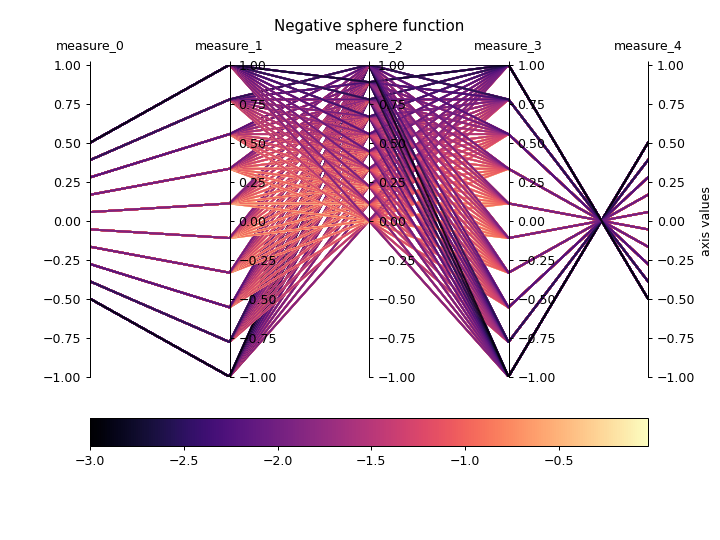# ribs.visualize.parallel_axes_plot¶

ribs.visualize.parallel_axes_plot(archive, ax=None, bc_order=None, cmap='magma', linewidth=1.5, alpha=0.8, vmin=None, vmax=None, sort_archive=False, cbar_orientation='horizontal', cbar_pad=0.1)[source]

Visualizes archive elites in behavior space with a parallel axes plot.

This visualization is meant to show the coverage of the behavior space at a glance. Each axis represents one behavioral dimension, and each line in the diagram represents one elite in the archive. Three main things are evident from this plot:

• Behavior space coverage, as determined by the amount of the axis that has lines passing through it. If the lines are passing through all parts of the axis, then there is likely good coverage for that BC.

• Correlation between neighboring BCs. In the below example, we see perfect correlation between behavior_0 and behavior_1, since none of the lines cross each other. We also see the perfect negative correlation between behavior_3 and behavior_4, indicated by the crossing of all lines at a single point.

• Whether certain values of the behavior dimensions affect the objective value strongly. In the below example, we see behavior_2 has many elites with high objective near zero. This is more visible when sort_archive is passed in, as elites with higher objective values will be plotted on top of individuals with lower objective values.

Examples

>>> import numpy as np
>>> import matplotlib.pyplot as plt
>>> from ribs.archives import GridArchive
>>> from ribs.visualize import parallel_axes_plot
>>> # Populate the archive with the negative sphere function.
>>> archive = GridArchive(
...               [20, 20, 20, 20, 20],
...               [(-1, 1), (-1, 1), (-1, 1), (-1, 1), (-1, 1)]
...           )
>>> archive.initialize(solution_dim=3)
>>> for x in np.linspace(-1, 1, 100):
...     for y in np.linspace(0, 1, 100):
...         for z in np.linspace(-1, 1, 100):
...                 solution=np.array([x,y,z]),
...                 objective_value=-(x**2 + y**2 + z**2),
...                 behavior_values=np.array([0.5*x,x,y,z,-0.5*z]),
...             )
>>> # Plot a heatmap of the archive.
>>> plt.figure(figsize=(8, 6))
>>> parallel_axes_plot(archive)
>>> plt.title("Negative sphere function")
>>> plt.ylabel("axis values")
>>> plt.show()Parameters
• archive (ArchiveBase) – Any ribs archive.

• ax (matplotlib.axes.Axes) – Axes on which to create the plot. If None, the current axis will be used.

• bc_order (list of int or list of (int, str)) – If this is a list of ints, it specifies the axes order for BCs (e.g. [2, 0, 1]). If this is a list of tuples, each tuple takes the form (int, str) where the int specifies the BC index and the str specifies a name for the BC (e.g. [(1, "y-value"), (2, "z-value"), (0, "x-value)]). The order specified does not need to have the same number of elements as the number of behaviors in the archive, e.g. [1, 3] or [1, 2, 3, 2].

• cmap (str, list, matplotlib.colors.Colormap) – Colormap to use when plotting intensity. Either the name of a colormap, a list of RGB or RGBA colors (i.e. an Nx3 or Nx4 array), or a colormap object.

• linewidth (float) – Line width for each elite in the plot.

• alpha (float) – Opacity of the line for each elite (passing a low value here may be helpful if there are many archive elites, as more elites would be visible).

• vmin (float) – Minimum objective value to use in the plot. If None, the minimum objective value in the archive is used.

• vmax (float) – Maximum objective value to use in the plot. If None, the maximum objective value in the archive is used.

• sort_archive (boolean) – if true, sorts the archive so that the highest performing elites are plotted on top of lower performing elites.

Warning

This may be slow for large archives.

• cbar_orientation (str) – The orientation of the colorbar. Use either 'vertical' or 'horizontal'

• cbar_pad (float) – The amount of padding to use for the colorbar.

Raises
• ValueErrorcbar_orientation has an invalid value.

• ValueError – The bcs provided do not exist in the archive.

• TypeError – bc_order is not a list of all ints or all tuples.